NameFlagsCard.TypeDescription & Constraints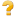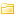Extension 0..*ExtensionGoals related to this Goal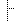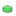extension:type 1..1Extensionpredecessor | successor | replacement | other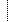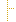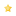extension 0..0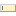url 1..1uri"type"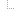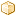value[x] 1..1CodeableConceptValue of extension
Binding: GoalRelationshipType (extensible): Types of relationships between two goals.extension:target 1..1ExtensionRelated goalextension 0..0url 1..1uri"target"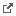value[x] 1..1Reference(Goal)Value of extensionurl 1..1uri"http://hl7.org/fhir/StructureDefinition/goal-relationship"value[x] 0..0Documentation for this format
<status value="active"/> <experimental value="false"/> <date value="2014-12-07"/> <publisher value="HL7 International / Patient Care"/> <contact> <telecom> <system value="url"/> <value value="http://www.hl7.org/Special/committees/patientcare"/> </telecom> </contact> <description value="Establishes a relationship between this goal and other goals."/> <jurisdiction> <coding> <system value="http://unstats.un.org/unsd/methods/m49/m49.htm"/> <code value="001"/> </coding> </jurisdiction> <fhirVersion value="5.0.0"/> <mapping> <identity value="rim"/> <uri value="http://hl7.org/v3"/> <name value="RIM Mapping"/> </mapping> <kind value="complex-type"/> <abstract value="false"/> <context> <type value="element"/> <expression value="Goal"/> </context> <context> <type value="element"/> <expression value="PlanDefinition.goal"/> </context> <type value="Extension"/> <baseDefinition value="http://hl7.org/fhir/StructureDefinition/Extension"/> <derivation value="constraint"/> <snapshot> <element id="Extension"> <path value="Extension"/> <short value="Goals related to this Goal"/> <definition value="Establishes a relationship between this goal and other goals."/> <min value="0"/> <max value="*"/> <base> <path value="Extension"/> <min value="0"/> <max value="*"/> </base> <constraint> <key value="ele-1"/> <severity value="error"/> <human value="All FHIR elements must have a @value or children"/> <expression value="hasValue() or (children().count() > id.count())"/> <source value="http://hl7.org/fhir/StructureDefinition/Element"/> </constraint> <constraint> <key value="ext-1"/> <severity value="error"/> <human value="Must have either extensions or value[x], not both"/> <expression value="extension.exists() != value.exists()"/> <source value="http://hl7.org/fhir/StructureDefinition/Extension"/> </constraint> <isModifier value="false"/> </element> <element id="Extension.id"> <path value="Extension.id"/> <representation value="xmlAttr"/> <short value="Unique id for inter-element referencing"/> <definition value="Unique id for the element within a resource (for internal references). This may be any string value that does not contain spaces."/> <min value="0"/> <max value="1"/> <base> <path value="Element.id"/> <min value="0"/> <max value="1"/> </base> <type> <extension url="http://hl7.org/fhir/StructureDefinition/structuredefinition-fhir-type"> <valueUrl value="id"/> </extension> <code value="http://hl7.org/fhirpath/System.String"/> </type> <condition value="ele-1"/> <isModifier value="false"/> <isSummary value="false"/> <mapping> <identity value="rim"/> <map value="n/a"/> </mapping> </element> <element id="Extension.extension"> <path value="Extension.extension"/> <slicing> <discriminator> <type value="value"/> <path value="url"/> </discriminator> <description value="Extensions are always sliced by (at least) url"/> <rules value="open"/> </slicing> <short value="Additional content defined by implementations"/> <definition value="May be used to represent additional information that is not part of the basic definition of the element. To make the use of extensions safe and managable, there is a strict set of governance applied to the definition and use of extensions. Though any implementer can define an extension, there is a set of requirements that SHALL be met as part of the definition of the extension."/> <comment value="There can be no stigma associated with the use of extensions by any application, project, or standard - regardless of the institution or jurisdiction that uses or defines the extensions. The use of extensions is what allows the FHIR specification to retain a core level of simplicity for everyone."/> <alias value="extensions"/> <alias value="user content"/> <min value="2"/> <max value="*"/> <base> <path value="Element.extension"/> <min value="0"/> <max value="*"/> </base> <type> <code value="Extension"/> </type> <constraint> <key value="ele-1"/> <severity value="error"/> <human value="All FHIR elements must have a @value or children"/> <expression value="hasValue() or (children().count() > id.count())"/> <source value="http://hl7.org/fhir/StructureDefinition/Element"/> </constraint> <constraint> <key value="ext-1"/> <severity value="error"/> <human value="Must have either extensions or value[x], not both"/> <expression value="extension.exists() != value.exists()"/> <source value="http://hl7.org/fhir/StructureDefinition/Extension"/> </constraint> <isModifier value="false"/> <isSummary value="false"/> <mapping> <identity value="rim"/> <map value="n/a"/> </mapping> </element> <element id="Extension.extension:type"> <path value="Extension.extension"/> <sliceName value="type"/> <short value="predecessor | successor | replacement | other"/> <definition value="Identifies what kind of relationship exists between source and target goal."/> <min value="1"/> <max value="1"/> <base> <path value="Element.extension"/> <min value="0"/> <max value="*"/> </base> <type> <code value="Extension"/> </type> <constraint> <key value="ele-1"/> <severity value="error"/> <human value="All FHIR elements must have a @value or children"/> <expression value="hasValue() or (children().count() > id.count())"/> <source value="http://hl7.org/fhir/StructureDefinition/Element"/> </constraint> <constraint> <key value="ext-1"/> <severity value="error"/> <human value="Must have either extensions or value[x], not both"/> <expression value="extension.exists() != value.exists()"/> <source value="http://hl7.org/fhir/StructureDefinition/Extension"/> </constraint> <isModifier value="false"/> <isSummary value="false"/> </element> <element id="Extension.extension:type.id"> <path value="Extension.extension.id"/> <representation value="xmlAttr"/> <short value="Unique id for inter-element referencing"/> <definition value="Unique id for the element within a resource (for internal references). This may be any string value that does not contain spaces."/> <min value="0"/> <max value="1"/> <base> <path value="Element.id"/> <min value="0"/> <max value="1"/> </base> <type> <extension url="http://hl7.org/fhir/StructureDefinition/structuredefinition-fhir-type"> <valueUrl value="id"/> </extension> <code value="http://hl7.org/fhirpath/System.String"/> </type> <condition value="ele-1"/> <isModifier value="false"/> <isSummary value="false"/> <mapping> <identity value="rim"/> <map value="n/a"/> </mapping> </element> <element id="Extension.extension:type.extension"> <path value="Extension.extension.extension"/> <slicing> <discriminator> <type value="value"/> <path value="url"/> </discriminator> <description value="Extensions are always sliced by (at least) url"/> <rules value="open"/> </slicing> <short value="Extension"/> <definition value="An Extension"/> <min value="0"/> <max value="0"/> <base> <path value="Element.extension"/> <min value="0"/> <max value="*"/> </base> <type> <code value="Extension"/> </type> <constraint> <key value="ele-1"/> <severity value="error"/> <human value="All FHIR elements must have a @value or children"/> <expression value="hasValue() or (children().count() > id.count())"/> <source value="http://hl7.org/fhir/StructureDefinition/Element"/> </constraint> <constraint> <key value="ext-1"/> <severity value="error"/> <human value="Must have either extensions or value[x], not both"/> <expression value="extension.exists() != value.exists()"/> <source value="http://hl7.org/fhir/StructureDefinition/Extension"/> </constraint> <isModifier value="false"/> <isSummary value="false"/> </element> <element id="Extension.extension:type.url"> <path value="Extension.extension.url"/> <representation value="xmlAttr"/> <short value="identifies the meaning of the extension"/> <definition value="Source of the definition for the extension code - a logical name or a URL."/> <comment value="The definition may point directly to a computable or human-readable definition of the extensibility codes, or it may be a logical URI as declared in some other specification. The definition SHALL be a URI for the Structure Definition defining the extension."/> <min value="1"/> <max value="1"/> <base> <path value="Extension.url"/> <min value="1"/> <max value="1"/> </base> <type> <code value="uri"/> </type> <fixedUri value="type"/> <isModifier value="false"/> <isSummary value="false"/> <mapping> <identity value="rim"/> <map value="N/A"/> </mapping> </element> <element id="Extension.extension:type.value[x]"> <path value="Extension.extension.value[x]"/> <short value="Value of extension"/> <definition value="Value of extension - must be one of a constrained set of the data types (see [Extensibility](http://hl7.org/fhir/R5/extensibility.html) for a list)."/> <min value="1"/> <max value="1"/> <base> <path value="Extension.value[x]"/> <min value="0"/> <max value="1"/> </base> <type> <code value="CodeableConcept"/> </type> <condition value="ext-1"/> <constraint> <key value="ele-1"/> <severity value="error"/> <human value="All FHIR elements must have a @value or children"/> <expression value="hasValue() or (children().count() > id.count())"/> <source value="http://hl7.org/fhir/StructureDefinition/Element"/> </constraint> <isModifier value="false"/> <isSummary value="false"/> <binding> <extension url="http://hl7.org/fhir/StructureDefinition/elementdefinition-bindingName"> <valueString value="GoalRelationshipType"/> </extension> <strength value="extensible"/> <description value="Types of relationships between two goals."/> <valueSet value="http://hl7.org/fhir/ValueSet/goal-relationship-type"/> </binding> <mapping> <identity value="rim"/> <map value="N/A"/> </mapping> </element> <element id="Extension.extension:target"> <path value="Extension.extension"/> <sliceName value="target"/> <short value="Related goal"/> <definition value="The goal the relationship exists with."/> <min value="1"/> <max value="1"/> <base> <path value="Element.extension"/> <min value="0"/> <max value="*"/> </base> <type> <code value="Extension"/> </type> <constraint> <key value="ele-1"/> <severity value="error"/> <human value="All FHIR elements must have a @value or children"/> <expression value="hasValue() or (children().count() > id.count())"/> <source value="http://hl7.org/fhir/StructureDefinition/Element"/> </constraint> <constraint> <key value="ext-1"/> <severity value="error"/> <human value="Must have either extensions or value[x], not both"/> <expression value="extension.exists() != value.exists()"/> <source value="http://hl7.org/fhir/StructureDefinition/Extension"/> </constraint> <isModifier value="false"/> <isSummary value="false"/> </element> <element id="Extension.extension:target.id"> <path value="Extension.extension.id"/> <representation value="xmlAttr"/> <short value="Unique id for inter-element referencing"/> <definition value="Unique id for the element within a resource (for internal references). This may be any string value that does not contain spaces."/> <min value="0"/> <max value="1"/> <base> <path value="Element.id"/> <min value="0"/> <max value="1"/> </base> <type> <extension url="http://hl7.org/fhir/StructureDefinition/structuredefinition-fhir-type"> <valueUrl value="id"/> </extension> <code value="http://hl7.org/fhirpath/System.String"/> </type> <condition value="ele-1"/> <isModifier value="false"/> <isSummary value="false"/> <mapping> <identity value="rim"/> <map value="n/a"/> </mapping> </element> <element id="Extension.extension:target.extension"> <path value="Extension.extension.extension"/> <slicing> <discriminator> <type value="value"/> <path value="url"/> </discriminator> <description value="Extensions are always sliced by (at least) url"/> <rules value="open"/> </slicing> <short value="Extension"/> <definition value="An Extension"/> <min value="0"/> <max value="0"/> <base> <path value="Element.extension"/> <min value="0"/> <max value="*"/> </base> <type> <code value="Extension"/> </type> <constraint> <key value="ele-1"/> <severity value="error"/> <human value="All FHIR elements must have a @value or children"/> <expression value="hasValue() or (children().count() > id.count())"/> <source value="http://hl7.org/fhir/StructureDefinition/Element"/> </constraint> <constraint> <key value="ext-1"/> <severity value="error"/> <human value="Must have either extensions or value[x], not both"/> <expression value="extension.exists() != value.exists()"/> <source value="http://hl7.org/fhir/StructureDefinition/Extension"/> </constraint> <isModifier value="false"/> <isSummary value="false"/> </element> <element id="Extension.extension:target.url"> <path value="Extension.extension.url"/> <representation value="xmlAttr"/> <short value="identifies the meaning of the extension"/> <definition value="Source of the definition for the extension code - a logical name or a URL."/> <comment value="The definition may point directly to a computable or human-readable definition of the extensibility codes, or it may be a logical URI as declared in some other specification. The definition SHALL be a URI for the Structure Definition defining the extension."/> <min value="1"/> <max value="1"/> <base> <path value="Extension.url"/> <min value="1"/> <max value="1"/> </base> <type> <code value="uri"/> </type> <fixedUri value="target"/> <isModifier value="false"/> <isSummary value="false"/> <mapping> <identity value="rim"/> <map value="N/A"/> </mapping> </element> <element id="Extension.extension:target.value[x]"> <path value="Extension.extension.value[x]"/> <short value="Value of extension"/> <definition value="Value of extension - must be one of a constrained set of the data types (see [Extensibility](http://hl7.org/fhir/R5/extensibility.html) for a list)."/> <min value="1"/> <max value="1"/> <base> <path value="Extension.value[x]"/> <min value="0"/> <max value="1"/> </base> <type> <code value="Reference"/> <targetProfile value="http://hl7.org/fhir/StructureDefinition/Goal"/> </type> <condition value="ext-1"/> <constraint> <key value="ele-1"/> <severity value="error"/> <human value="All FHIR elements must have a @value or children"/> <expression value="hasValue() or (children().count() > id.count())"/> <source value="http://hl7.org/fhir/StructureDefinition/Element"/> </constraint> <isModifier value="false"/> <isSummary value="false"/> <mapping> <identity value="rim"/> <map value="N/A"/> </mapping> </element> <element id="Extension.url"> <path value="Extension.url"/> <representation value="xmlAttr"/> <short value="identifies the meaning of the extension"/> <definition value="Source of the definition for the extension code - a logical name or a URL."/> <comment value="The definition may point directly to a computable or human-readable definition of the extensibility codes, or it may be a logical URI as declared in some other specification. The definition SHALL be a URI for the Structure Definition defining the extension."/> <min value="1"/> <max value="1"/> <base> <path value="Extension.url"/> <min value="1"/> <max value="1"/> </base> <type> <extension url="http://hl7.org/fhir/StructureDefinition/structuredefinition-fhir-type"> <valueUrl value="uri"/> </extension> <code value="http://hl7.org/fhirpath/System.String"/> </type> <fixedUri value="http://hl7.org/fhir/StructureDefinition/goal-relationship"/> <isModifier value="false"/> <isSummary value="false"/> <mapping> <identity value="rim"/> <map value="N/A"/> </mapping> </element> <element id="Extension.value[x]"> <path value="Extension.value[x]"/> <short value="Value of extension"/> <definition value="Value of extension - must be one of a constrained set of the data types (see [Extensibility](http://hl7.org/fhir/R5/extensibility.html) for a list)."/> <min value="0"/> <max value="0"/> <base> <path value="Extension.value[x]"/> <min value="0"/> <max value="1"/> </base> <type> <code value="base64Binary"/> </type> <type> <code value="boolean"/> </type> <type> <code value="canonical"/> </type> <type> <code value="code"/> </type> <type> <code value="date"/> </type> <type> <code value="dateTime"/> </type> <type> <code value="decimal"/> </type> <type> <code value="id"/> </type> <type> <code value="instant"/> </type> <type> <code value="integer"/> </type> <type> <code value="integer64"/> </type> <type> <code value="markdown"/> </type> <type> <code value="oid"/> </type> <type> <code value="positiveInt"/> </type> <type> <code value="string"/> </type> <type> <code value="time"/> </type> <type> <code value="unsignedInt"/> </type> <type> <code value="uri"/> </type> <type> <code value="url"/> </type> <type> <code value="uuid"/> </type> <type> <code value="Address"/> </type> <type> <code value="Age"/> </type> <type> <code value="Annotation"/> </type> <type> <code value="Attachment"/> </type> <type> <code value="CodeableConcept"/> </type> <type> <code value="CodeableReference"/> </type> <type> <code value="Coding"/> </type> <type> <code value="ContactPoint"/> </type> <type> <code value="Count"/> </type> <type> <code value="Distance"/> </type> <type> <code value="Duration"/> </type> <type> <code value="HumanName"/> </type> <type> <code value="Identifier"/> </type> <type> <code value="Money"/> </type> <type> <code value="Period"/> </type> <type> <code value="Quantity"/> </type> <type> <code value="Range"/> </type> <type> <code value="Ratio"/> </type> <type> <code value="RatioRange"/> </type> <type> <code value="Reference"/> </type> <type> <code value="SampledData"/> </type> <type> <code value="Signature"/> </type> <type> <code value="Timing"/> </type> <type> <code value="ContactDetail"/> </type> <type> <code value="DataRequirement"/> </type> <type> <code value="Expression"/> </type> <type> <code value="ParameterDefinition"/> </type> <type> <code value="RelatedArtifact"/> </type> <type> <code value="TriggerDefinition"/> </type> <type> <code value="UsageContext"/> </type> <type> <code value="Availability"/> </type> <type> <code value="ExtendedContactDetail"/> </type> <type> <code value="Dosage"/> </type> <type> <code value="Meta"/> </type> <condition value="ext-1"/> <constraint> <key value="ele-1"/> <severity value="error"/> <human value="All FHIR elements must have a @value or children"/> <expression value="hasValue() or (children().count() > id.count())"/> <source value="http://hl7.org/fhir/StructureDefinition/Element"/> </constraint> <isModifier value="false"/> <isSummary value="false"/> <mapping> <identity value="rim"/> <map value="N/A"/> </mapping> </element> </snapshot> <differential> <element id="Extension"> <path value="Extension"/> <short value="Goals related to this Goal"/> <definition value="Establishes a relationship between this goal and other goals."/> <min value="0"/> <max value="*"/> </element> <element id="Extension.extension:type"> <path value="Extension.extension"/> <sliceName value="type"/> <short value="predecessor | successor | replacement | other"/> <definition value="Identifies what kind of relationship exists between source and target goal."/> <min value="1"/> <max value="1"/> <type> <code value="Extension"/> </type> </element> <element id="Extension.extension:type.extension"> <path value="Extension.extension.extension"/> <max value="0"/> </element> <element id="Extension.extension:type.url"> <path value="Extension.extension.url"/> <type> <code value="uri"/> </type> <fixedUri value="type"/> </element> <element id="Extension.extension:type.value[x]"> <path value="Extension.extension.value[x]"/> <min value="1"/> <type> <code value="CodeableConcept"/> </type> <binding> <extension url="http://hl7.org/fhir/StructureDefinition/elementdefinition-bindingName"> <valueString value="GoalRelationshipType"/> </extension> <strength value="extensible"/> <description value="Types of relationships between two goals."/> <valueSet value="http://hl7.org/fhir/ValueSet/goal-relationship-type"/> </binding> </element> <element id="Extension.extension:target"> <path value="Extension.extension"/> <sliceName value="target"/> <short value="Related goal"/> <definition value="The goal the relationship exists with."/> <min value="1"/> <max value="1"/> <type> <code value="Extension"/> </type> </element> <element id="Extension.extension:target.extension"> <path value="Extension.extension.extension"/> <max value="0"/> </element> <element id="Extension.extension:target.url"> <path value="Extension.extension.url"/> <type> <code value="uri"/> </type> <fixedUri value="target"/> </element> <element id="Extension.extension:target.value[x]"> <path value="Extension.extension.value[x]"/> <min value="1"/> <type> <code value="Reference"/> <targetProfile value="http://hl7.org/fhir/StructureDefinition/Goal"/> </type> </element> <element id="Extension.url"> <path value="Extension.url"/> <fixedUri value="http://hl7.org/fhir/StructureDefinition/goal-relationship"/> </element> <element id="Extension.value[x]"> <path value="Extension.value[x]"/> <min value="0"/> <max value="0"/> </element> </differential> </StructureDefinition>